# SPSS tutorials

BASICS DATA ANALYSIS T-TEST ANOVA CHI-SQUARE TEST

# SPSS Simple Linear Regression Tutorial

## Research Question and Data

Company X had 10 employees take an IQ and job performance test. The resulting data -part of which are shown below- are in simple-linear-regression.sav.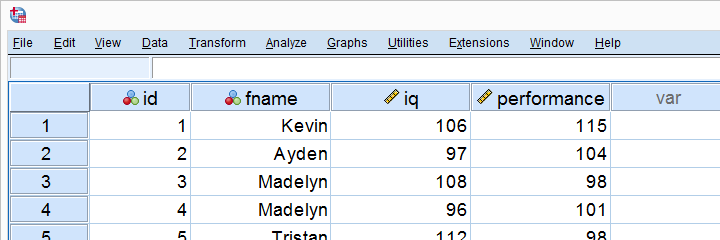The main thing Company X wants to figure out is does IQ predict job performance? And -if so- how? We'll answer these questions by running a simple linear regression analysis in SPSS.

## Create Scatterplot with Fit Line

A great starting point for our analysis is a scatterplot. This will tell us if the IQ and performance scores and their relation -if any- make any sense in the first place. We'll create our chart from GraphsLegacy DialogsScatter/Dot and we'll then follow the screenshots below.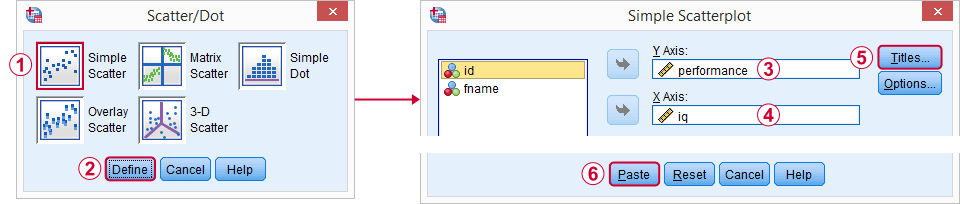I personally like to throw in

• a title that says what my audience are basically looking at and
• a subtitle that says which respondents or observations are shown and how many.

Walking through the dialogs resulted in the syntax below. So let's run it.

## SPSS Scatterplot with Titles Syntax

*Scatterplot with title and subtitle from Graphs -> Legacy Dialogs -> Scatter.

GRAPH
/SCATTERPLOT(BIVAR)=iq WITH performance
/MISSING=LISTWISE
/TITLE='Scatterplot Performance with IQ'
/subtitle 'All Respondents | N = 10'.

## Result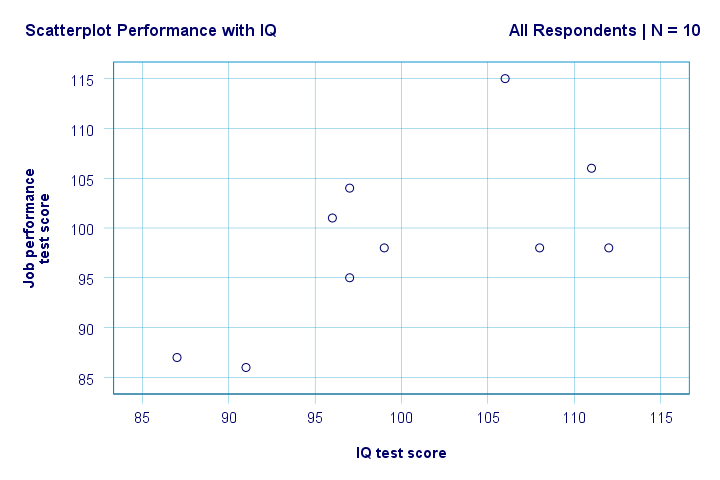Right. So first off, we don't see anything weird in our scatterplot. There seems to be a moderate correlation between IQ and performance: on average, respondents with higher IQ scores seem to be perform better. This relation looks roughly linear.
Let's now add a regression line to our scatterplot. Right-clicking it and selecting Edit contentIn Separate Window opens up a Chart Editor window. Here we simply click the “Add Fit Line at Total” icon as shown below.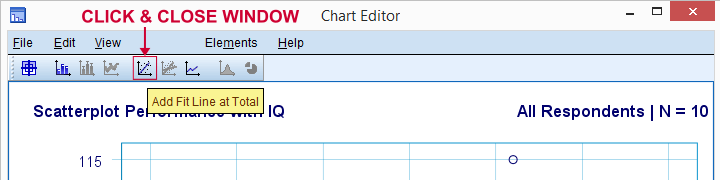By default, SPSS now adds a linear regression line to our scatterplot. The result is shown below.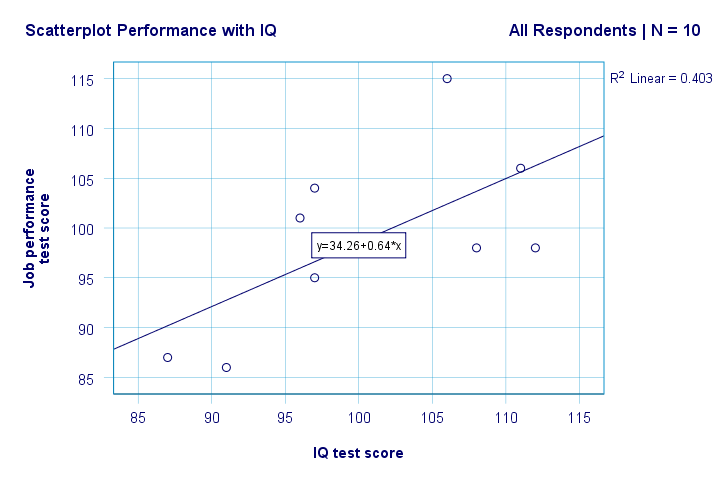We now have some first basic answers to our research questions. R2 = 0.403 indicates that IQ accounts for some 40.3% of the variance in performance scores. That is, IQ predicts performance fairly well in this sample.
But how can we best predict job performance from IQ? Well, in our scatterplot y is performance (shown on the y-axis) and x is IQ (shown on the x-axis). So that'll be performance = 34.26 + 0.64 * IQ. So for a job applicant with an IQ score of 115, we'll predict 34.26 + 0.64 * 115 = 107.86 as his/her most likely future performance score.
Right, so that gives us a basic idea about the relation between IQ and performance and presents it visually. However, a lot of information -statistical significance and confidence intervals- is still missing. So let's go and get it.

## SPSS Linear Regression Dialogs

Rerunning our minimal regression analysis from AnalyzeRegressionLinear gives us much more detailed output. The screenshots below show how we'll proceed.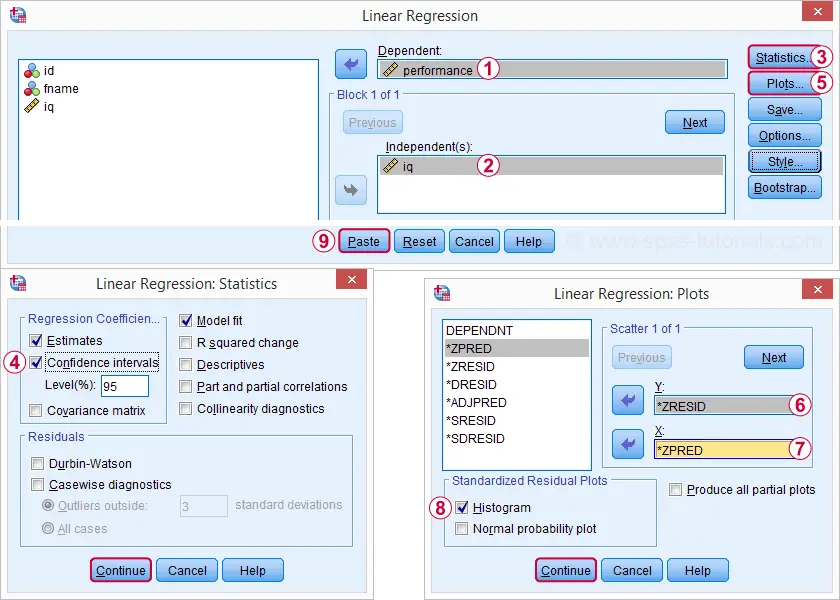Selecting these options results in the syntax below. Let's run it.

## SPSS Simple Linear Regression Syntax

*Simple regression with residual plots and confidence intervals.

REGRESSION
/MISSING LISTWISE
/STATISTICS COEFF OUTS CI(95) R ANOVA
/CRITERIA=PIN(.05) POUT(.10)
/NOORIGIN
/DEPENDENT performance
/METHOD=ENTER iq
/SCATTERPLOT=(*ZRESID ,*ZPRED)
/RESIDUALS HISTOGRAM(ZRESID).

## SPSS Regression Output I - Coefficients

Unfortunately, SPSS gives us much more regression output than we need. We can safely ignore most of it. However, a table of major importance is the coefficients table shown below.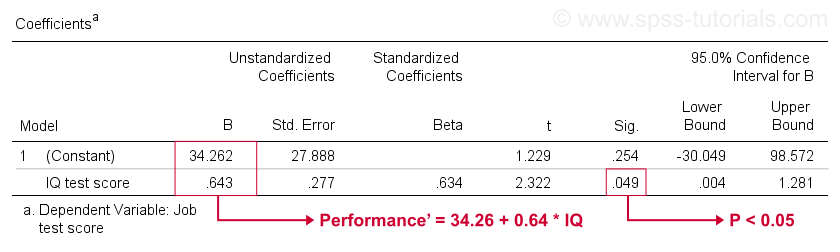This table shows the B-coefficients we already saw in our scatterplot. As indicated, these imply the linear regression equation that best estimates job performance from IQ in our sample.
Second, remember that we usually reject the null hypothesis if p < 0.05. The B coefficient for IQ has “Sig” or p = 0.049. It's statistically significantly different from zero.
However, its 95% confidence interval -roughly, a likely range for its population value- is [0.004,1.281]. So B is probably not zero but it may well be very close to zero. The confidence interval is huge -our estimate for B is not precise at all- and this is due to the minimal sample size on which the analysis is based.

## SPSS Regression Output II - Model Summary

Apart from the coefficients table, we also need the Model Summary table for reporting our results.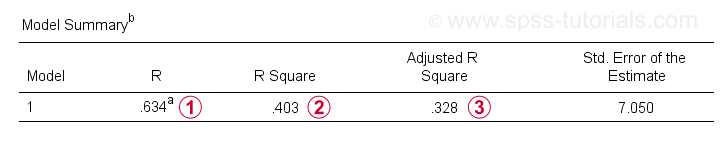R is the correlation between the regression predicted values and the actual values. For simple regression, R is equal to the correlation between the predictor and dependent variable.R Square -the squared correlation- indicates the proportion of variance in the dependent variable that's accounted for by the predictor(s) in our sample data.Adjusted R-square estimates R-square when applying our (sample based) regression equation to the entire population.

Adjusted r-square gives a more realistic estimate of predictive accuracy than simply r-square. In our example, the large difference between them -generally referred to as shrinkage- is due to our very minimal sample size of only N = 10.

In any case, this is bad news for Company X: IQ doesn't really predict job performance so nicely after all.

## Evaluating the Regression Assumptions

The main assumptions for regression are

1. Independent observations;
2. Normality: errors must follow a normal distribution in population;
3. Linearity: the relation between each predictor and the dependent variable is linear;
4. Homoscedasticity: errors must have constant variance over all levels of predicted value.

1. If each case (row of cells in data view) in SPSS represents a separate person, we usually assume that these are “independent observations”. Next, assumptions 2-4 are best evaluated by inspecting the regression plots in our output.

2. If normality holds, then our regression residuals should be (roughly) normally distributed. The histogram below doesn't show a clear departure from normality.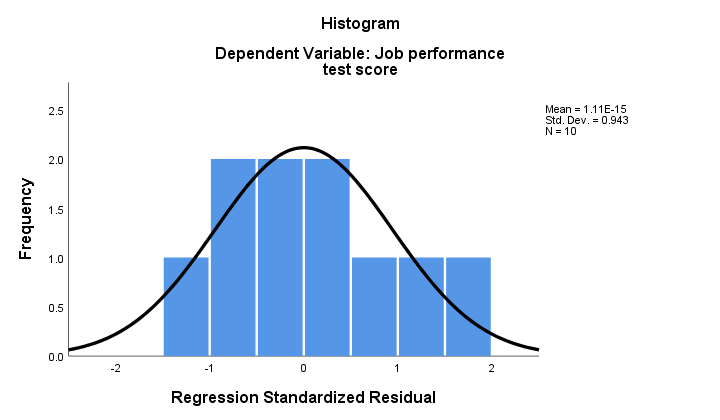The regression procedure can add these residuals as a new variable to your data. By doing so, you could run a Kolmogorov-Smirnov test for normality on them. For the tiny sample at hand, however, this test will hardly have any statistical power. So let's skip it.

The 3. linearity and 4. homoscedasticity assumptions are best evaluated from a residual plot. This is a scatterplot with predicted values in the x-axis and residuals on the y-axis as shown below. Both variables have been standardized but this doesn't affect the shape of the pattern of dots.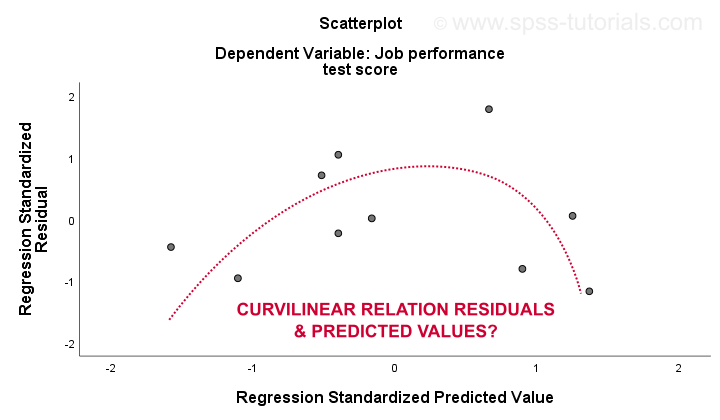Honestly, the residual plot shows strong curvilinearity. I manually drew the curve that I think fits best the overall pattern. Assuming a curvilinear relation probably resolves the heteroscedasticity too but things are getting way too technical now. The basic point is simply that some assumptions don't hold. The most common solutions for these problems -from worst to best- are

• ignoring these assumptions altogether;
• lying that the regression plots don't indicate any violations of the model assumptions;
• a non linear transformation -such as logarithmic- to the dependent variable;
• fitting a curvilinear model -which we'll give a shot in a minute.

## APA Guidelines for Reporting Regression

The figure below is -quite literally- a textbook illustration for reporting regression in APA format.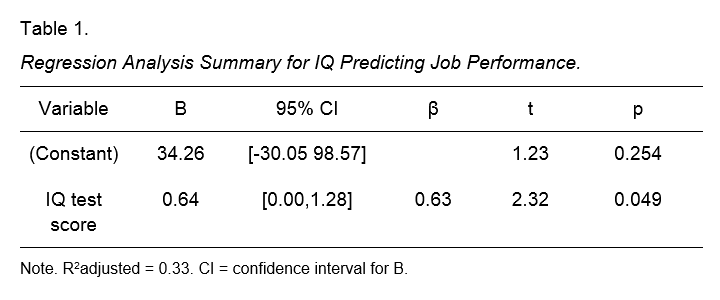Creating this exact table from the SPSS output is a real pain in the ass. Editing it goes easier in Excel than in WORD so that may save you a at least some trouble.
Alternatively, try to get away with copy-pasting the (unedited) SPSS output and pretend to be unaware of the exact APA format.

## Non Linear Regression Experiment

Our sample size is too small to really fit anything beyond a linear model. But we did so anyway -just curiosity. The easiest option in SPSS is under AnalyzeRegressionCurve Estimation. We're not going to discuss the dialogs but we pasted the syntax below.

## SPSS Non Linear Regression Syntax

*Non linear regression from Analyze - Regression - Curve Estimation.

TSET NEWVAR=NONE.
CURVEFIT
/VARIABLES=performance WITH iq
/CONSTANT
/PLOT FIT.

## Results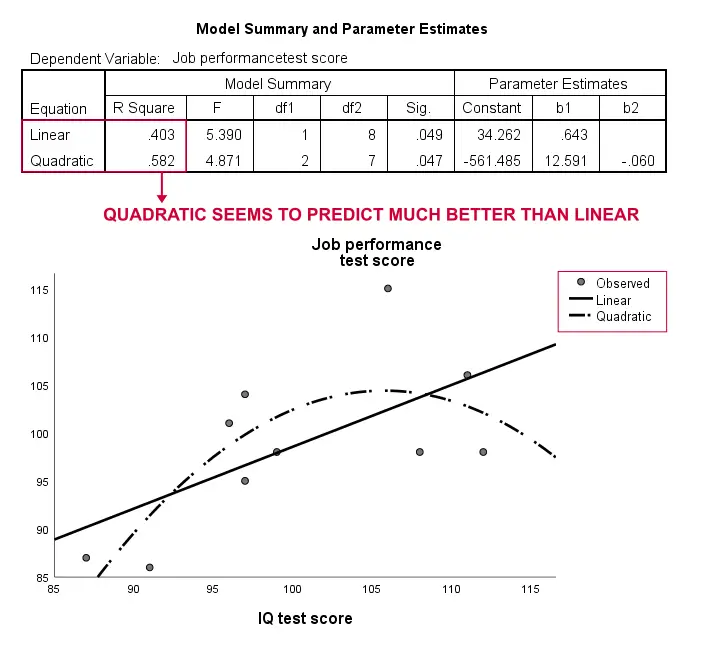Again, our sample is way too small to conclude anything serious. However, the results do kinda suggest that a curvilinear model fits our data much better than the linear one. We won't explore this any further but we did want to mention it; we feel that curvilinear models are routinely overlooked by social scientists.

# Let me know what you think!

*Required field. Your comment will show up after approval from a moderator.

# This tutorial has 5 comments

•### By Ruben Geert van den Berg on August 30th, 2019

Hi Omer!

The F-value reported by SPSS regression is pretty worthless. It only tells whether the entire regression model accounts for any variance at all. Not interesting.

Beta and p ("Sig.") are totally different. Beta says "how strong" a predictor predicts the outcome variable. P for any predictor indicates how likely it is that it doesn't account for any unique variance at all. These are different but complementary pieces of information. We therefore usually report both (and some other statistics too).

Hope that helps!

•### By Omer on August 30th, 2019

What about F value in reporting ?
Isn't Beta and P value has same meaning so is it necessary to report both value in table ?

•### By Emmy charles on July 21st, 2019

this knowledge is very helpful for me ...i get something from this ...thank you

•### By MUHAMMED TAHIR MUHAMMED on April 12th, 2019

Thanks for this great knowledge. It was useful

•### By Abdullahi Aden on March 12th, 2019

Very excellent Presentation on data analysis